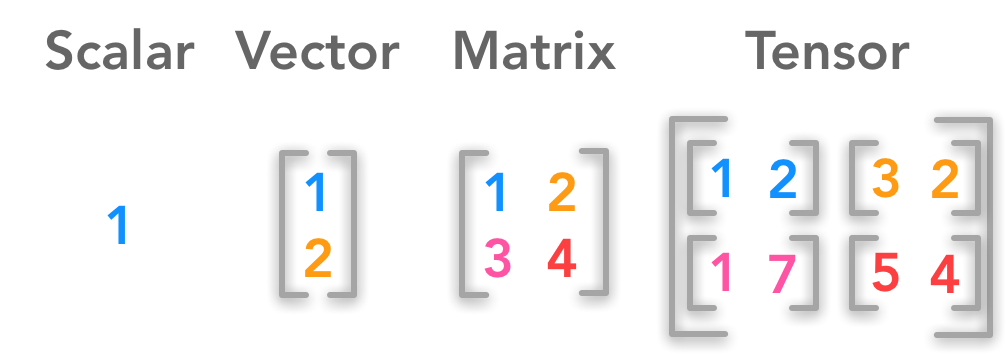# WTF is a Tensor?

Basically:

A tensor is a container which can house data in N dimensions, along with its linear operations, though there is nuance in what tensors technically are and what we refer to as tensors in practice.

and,

Mathematically speaking, tensors are more than simply a data container, however. Aside from holding numeric data, tensors also include descriptions of the valid linear transformations between tensors. Examples of such transformations, or relations, include the cross product and the dot product. From a computer science perspective, it can be helpful to think of tensors as being objects in an object-oriented sense, as opposed to simply being a data structure.Essentially a Scalar is a 0D Tensor, a Vector a 1D Tensor, a Matrix a 2D Tensor, and a Tensor a 3D Tensor.